NEET  >  Short & Long Answer Questions: Mechanical Properties of Solids

# Short & Long Answer Questions: Mechanical Properties of Solids - Notes | Study Physics Class 11 - NEET

 1 Crore+ students have signed up on EduRev. Have you?

Q.1 What is the nature of intermolecular forces?

Answer: Generally the intermolecular forces are attractive, but they are repulsive for intermolecular separations less than 10−10m.
Q.2 What is the origin of interatomic force?

Answer: Interatomic force arises due to the electrostatic interaction between the nuclei of two atoms, their electron clouds and between the nucleus of one atom and the electron cloud of the other atom.
Q.3 What is the origin of intermolecular force?

Answer: Intermolecular force arises due to the electrostatic interaction between the opposite charged ends of molecular dipoles.
Q.4 Are the intermolecular forces involved in the formation of liquids and solids different in nature? If yes, how?

Answer: Yes. The intermolecular forces involved in the formation of liquids are attractive in nature while in the formation of solids, the repulsive intermolecular forces are more important.
Q.5 State the factors due to which three states of matter differ from each other.

Answer: The three states of matter differ from each other due to the following two factors:

(i) The different magnitudes of inter-atomic and inter-molecular forces.

(ii) The degree of random thermal motion of the atoms and the molecules of a substance depending on temperature.
Q.6 What do you mean by long range order in a crystalline structure?

Answer: Long range order means that similar patterns of atoms or molecules repeat over a large distance in a crystal.
Q.7 What is the important structural difference between crystalline and glassy solids?

Answer: In crystalline solids the atoms or molecules are arranged in a definite and long range order, but in glassy solids there exists no such long range order in the arrangement of atoms or molecules.
Q.8 Amorphous solids do not melt at a sharp temperature, rather these have softening range. Explain this observation.

Answer: All bonds in an amorphous solid are not equally strong. When the solid is heated, weaker bonds get ruptured at lower temperatures and the stronger ones at higher temperatures. So the solid first softens and then finally melts.
Q.9 In what respect, the behaviour of glassy solids is similar to that of the liquids?

Answer: In case of glassy solids, the orderly arrangement of atoms is limited to a very short range and in this respect they are similar to liquids.
Q.10 Why do crystalline solids have well defined geometrical external shapes?

Answer: This is because the atoms and molecules are arranged in a definite geometrical repeating manner throughout the body of the crystal.
Q.11 Amorphous solids are not true solids. Why and what are they called then?

Answer: Like liquids, amorphous solids have disordered arrangement of atoms or molecules. The molecules of a liquid are free to move but the molecules of an amorphous solid are almost fixed at their positions i.e., amorphous solids are rigid due to their high viscosity. That is why, we say amorphous solids are super-cooled liquids of high viscosity.
Q.12 Our knowledge about crystalline solids is better than amorphous solids. Why?

Answer: As crystalline solids possess long range and regular arrangement of atoms, hence their behaviour can be easily understood.
Q.13 Crystalline solids are called true solids. Why?

Answer: This is because crystalline solids have well defined, regularly repeated three-dimensional arrangement of atoms or molecules.
Q.14 What is a perfectly elastic body? Give an example.

Answer: If, on removal of deforming force, a body completely regains its original configuration, then it is said to be perfectly elastic. For example, quartz.
Q.15 What is a perfectly plastic body? Give an example.

Answer: If, on removal of deforming force, a body does not regain its original configuration even a little, then it is said to be perfectly plastic. For example, putty.
Q.16 No material is perfectly elastic. Why?

Answer: All materials undergo a change in their original state, howsoever small it may be, after the removal of deforming force. Hence, there is no such material which is perfectly elastic.
Q.17 When does a body acquire a permanent set?

Answer: When the deforming force exceeds the elastic limit, the body acquires the permanent set.

Q.18 A thick wire is suspended from a rigid support, but no load is attached to its free end. Is this wire under stress?

Answer: Yes, the wire is under stress due to its own weight.
Q.19 State the two factors on which the modulus of elasticity depends.

Answer: The modulus of elasticity depends upon nature of the material and (ii) type of stress used in producing the strain.

Q.20 Is it possible to double the length of a metallic wire by applying a force over it?

Answer: No, it is not possible because within elastic limit strain is only of the order of 10−3. Wires actually break much before it is stretched to double the length.
Q.21 Is elastic limit a property of the material of the wire?

Q.22 Stress and pressure are both forces per unit area. Then in what respect does stress differ from pressure?

Answer: Pressure is the external force per unit area, while stress is the internal restoring force which comes into play in a deformed body acting transversely per unit area of the body.

Q.23 Among solids, liquids and gases, which can have all the three moduli of elasticity?

Answer: Only solids. Liquids and gases have only bulk modulus.
Q.24 Among solids, liquids and gases, which possess the greatest bulk modulus?

Q.25 Which type of elasticity is involved in the following cases? (i) Compressing of gas (ii) Compressing a liquid (iii) Stretching a wire (iv) Tangential push on the upper face of a block.

(ii) Bulk modulus

(iii) Young's modulus

(iv) Modulus of rigidity.
Q.26 What does the slope of stress versus strain graph give?

Answer: The slope of stress-strain gives modulus of elasticity.
Q.27 How does Young's modulus change with the rise of temperature?

Answer: Young's modulus decreases with the rise of temperature.
Q.28 Write copper, steel, glass and rubber in the order of increasing coefficient of elasticity.

Answer: Rubber < glass < copper < steel.
Q.29 Which is more elastic-water or air?

Answer: Water is more elastic than air. Air can be easily compressed while water is incompressible and bulk modulus is reciprocal of compressibility.
Q.30 Why are springs made of steel and not of copper?

Answer: Young's modulus of steel is greater than that of copper. So steel spring is stretched lesser than a copper spring under the same deforming force. Moreover, steel returns to its original state more quickly than copper on the removal of deforming force.
Q.31 In stretching a wire, we have to perform work. Why?

Answer: When a wire is stretched, inter-atomic forces of attraction come into play. In order to stretch the wire, work has to be done against these forces.
Q.32 What happens to the work done in stretching a wire?

Answer: The work done in stretching a wire is stored in it as elastic potential energy.
Q.33 Two identical springs of steel and copper are equally stretched. On which more work will have to be done?

Answer: Young's modulus of steel is greater than that of copper. In order to produce same extension, large force will have to be applied on the steel spring than that on the copper spring. Hence more work will be done on the steel spring.
Q.34  If two identical springs of steel and copper are pulled by applying equal forces, then in which case more work will have to be done?

Answer: Steel spring will be stretched to a lesser extent. Now more work will be done on the copper spring.
Q.35 Why does a wire get heated when it is bent back and forth?

Answer: When a wire is bent back and forth, its deformations are beyond elastic limit. The work done against interatomic forces is no longer stored totally in the form of potential energy. The crystalline structure of the wire gets affected and work done is converted into heat energy.

Q.36 A hard wire is broken by bending it repeatedly in alternating directions. Why?

Answer: When the wire is subjected to repeated alternating strains, the strength of its material decreases and the wire breaks.
Q.37 Why is the longer side of cross-section of girder used as depth?

Answer: Depression,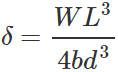Clearly, the depression of the girder will be small when depth d is large, because δ∝d−3.
Q.38 The ratio stress/strain remains constant for a small deformation. What happens to this ratio if deformation is made very large?

Answer: When the deforming force exceeds the elastic limit, the strain increases more rapidly than stress. Hence the ratio of stress/strain decreases.

Q.39 Why are electric poles given hollow structure?

Answer: This is because a hollow shaft is stronger than a solid shaft made from the same and equal amount of material.

Q.40 A wire fixed at the upper end stretches by length l by applying a force F. What is the work done in stretching the wire?

Answer: Work done in stretching the wire,
W=1/2 Stretching force × increase in length = 1/2 Fl.
Q.41  A wire suspended vertically from one of its ends is stretched by attaching a weight of 200 N to the lower end. The weight stretches the wire by 1 mm. Find the elastic energy stored in the wire.

Answer: Here F=200N,l=1mm=10−3m Elastic potential energy stored in the wire, U wire,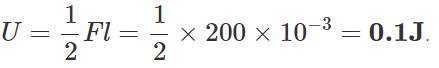Q.42 A wire stretches by a certain amount under a load. If the load and radius both are increased to four times, find the stretch caused in the wire.
Young's modulus,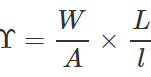∴ Elongation, l=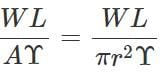When both load and radius are increased to four times, the elongation becomes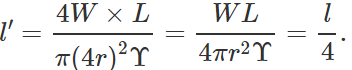Q.43 Crystalline solids have sharp melting points. Amorphous solids do not melt at a sharp temperature; rather these have a softening range of temperature. Explain.

Answer: All bonds in a crystalline solid are equally strong. When the solid is heated, these bonds ruptured at the same temperature. So crystalline solids have sharp melting points.  On the other hand, all bonds in an amorphous solid are not equally strong. When the solid is heated, weaker bonds break at lower temperatures and the stronger ones at higher temperatures. So the solid first softens and then finally melts, i.e., the amorphous solids do not have sharp melting points.
Q.44 Which is more elastic-rubber or steel? Explain.

Answer: Consider two rods of steel and rubber, each having length l and area of cross-section A. If they are subjected to the same deforming force F, then the extension Δlproduced in the steel rod will be less than the extension Δlr in the rubber rod, i.e.,
Δls<Δlr. Now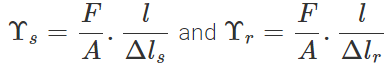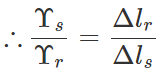As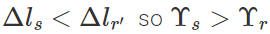i.e., Young's modulus for steel is greater than that of rubber. Hence steel is more elastic than rubber.
Q.45 Read each of the statements below care- fully and state, with reasons, if it is true or false. (a) When a material is under tensile stress, the restoring forces are caused by interatomic attraction while under compressional stress, the restoring forces are due to inter-atomic repulsion. (b) A piece of rubber under an ordinary stress can display 1000% strain: yet when unloaded returns to its original length. This shows that the elastic restoring forces in a rubber piece are strictly conservative. (c) Elastic restoring forces are strictly conservative only when Hooke's law is obeyed.

Answer: (a) True. In tensile stress, the interatomic separation becomes greater than equilibrium separation and the interatomic forces are attractive. In compressional stress, the interatomic separation becomes less than r0  and the interatomic forces are repulsive. (b) False. As the piece of rubber returns to its original length when unloaded, it is a case of elastic hysteresis in which there is some loss of energy. This signifies non-conservative forces. (c) False. Even if the stress-strain curves are non-linear, the elastic forces are conservative as long as loading and unloading curves are identical.
Q.46 Two wires of different materials are suspended from a rigid support. They have the same length and diameter and carry the same load at their free ends. (a) Will the stress and strain in each wire be the same? (b) Will the extension in both wires be the same?

Answer: (a) Stress in both the wires is the same as both the wires have the same diameter and carry the same load at their free ends. Strain will be different in the two wires as the wires are of different materials, even though the stress is the same.

(b) Because the original lengths of the two wires are equal and strains produced in them are different, hence extensions in the two wires will not be same.
Q.47 A cable is replaced by another of the same length and material but of twice the diameter. (a) How does this affect its elongation under a given load? (b) How many times will be the maximum load it can now support without exceeding the elastic limit?

Answer: (a) Young's modulus,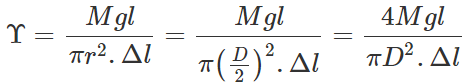where D is the diameter of the wire. Elongation,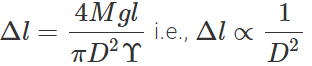Clearly, if the diameter is doubled, the elongation will become one-fourth.  (b) Also load,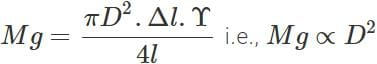Clearly, if the diameter is doubled, the wire can support 4 times the original load.
Q.48 A uniform plank of Young's modulus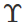is moved over a smooth horizontal surface by a constant horizontal force F. The area of transverse section of the plank is A. Find the compressive strain on the plank in the direction of the force.

Answer: As the force at the other end of the plank is zero, so the average stretching force =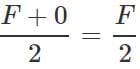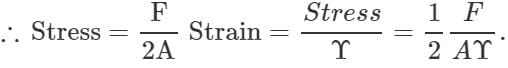Q.49 What are the factors which affect the elasticity of a material?

Answer: The following factors affect the elasticity of a material:

(i)Hammering and rolling. In both of these processes, the crystal grains are broken into small units and the elasticity of the material increases.

(ii) Annealing. This process results in the formation of larger crystal grains and elasticity of the material decreases.

(iii) Presence of impurities. Depending on the nature of the impurity, the elasticity of a material can be increased or decreased.

(iv) Temperature. Elasticity of most of the materials decreases with the increase in temperature. The elasticity of in var is not affected by temperature.
Q.50 Elasticity has a different meaning in physics than that in daily life. Comment.

Answer: In daily life, a body is said to be elastic if a large deformation or strain is produced on applying a given stress on it. In physics, elasticity is the property of the material of a body by virtue of which it opposes any. change in its size or shape when a stress is  applied on it. Thus a body will be more elastic if a small strain is produced on applying a given stress on it.
Q.51 Why a spring balance does not give correct measurement, when it has been used for a long time?

Answer: When a spring balance has been used for a long time, it develops an elastic fatigue, the spring of such a balance takes longer time to recover its original configuration and therefore it does not give correct measurement.
Q.52 Why the bridges are declared unsafe after a long use?

Answer: During its long use, a bridge suffers alternating strains continuously. Consequently, the elastic strength of the bridge gets reduced. After a long time, the bridge develops elastic fatigue and there occurs a permanent change in its structure. This permanent change ultimately leads the bridge to collapse. In order to avoid this event, the bridges are declared unsafe after long use.
Q.53 Two identical solid balls, one of ivory and the other of wet-clay, are dropped from the same height on the floor. Which will rise to a greater height after striking the floor and why?

Answer: The ball which is more elastic rises to a greater height after striking the floor. Ivory is more elastic than wet-clay. Hence the ball of ivory will rise to a greater height. In fact, the ball of wet-clay will not rise at all, it will get flattened.
Q.54 The breaking force for a wire is F. What will be the breaking force for (a) two parallel wires of the same size (b) for a single wire of double the thickness?

Answer: (a) When two wires of same size are suspended in parallel, a force F equal to the breaking force will act on each wire if a breaking force of 2F is applied on the parallel combination.

(b)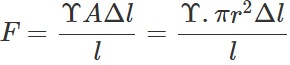i.e., F∝rThus for a single wire of double the thickness, the breaking force will be 4F.
Q.55 Graphite consists of planes of carbon atoms. Between atoms in the planes there are only weak forces. What kind of elastic properties do you expect from graphite?

Answer: Due to weak attractive forces between carbon atoms of different planes, it is easier to produce a large shearing strain by moving one plane of atoms over the other with the application of a small tangential stress. Now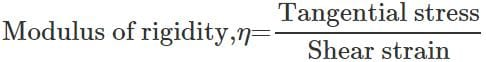Hence graphite should possess a small modulus of rigidity.

Q.56 Why does modulus of elasticity of most of the materials decrease with the increase of temperature?

Answer: As the temperature increases, the inter- atomic forces of attraction become weaker. For given stress, a larger strain or deformation is produced at a higher temperature. Hence the modulus of elasticity (stress/strain) decreases with the increase of temperature.

The document Short & Long Answer Questions: Mechanical Properties of Solids - Notes | Study Physics Class 11 - NEET is a part of the NEET Course Physics Class 11.
All you need of NEET at this link: NEET

## Physics Class 11

127 videos|464 docs|210 tests

## Physics Class 11

127 videos|464 docs|210 tests

### How to Prepare for NEET

Read our guide to prepare for NEET which is created by Toppers & the best Teachers

Track your progress, build streaks, highlight & save important lessons and more!

,

,

,

,

,

,

,

,

,

,

,

,

,

,

,

,

,

,

,

,

,

;